• 初等矩阵左乘 右乘与初等变换 矩阵左乘一个初等矩阵，相当于对矩阵进行了出的那个行变换，矩阵右乘一个初等矩阵，相当于对矩阵做了初等列变换，文章来源：...
初等矩阵左乘 右乘与初等变换
矩阵左乘一个初等矩阵，相当于对矩阵进行了出的那个行变换，矩阵右乘一个初等矩阵，相当于对矩阵做了初等列变换，文章来源：https://blog.csdn.net/silence1214/article/details/8634664
Latex排版的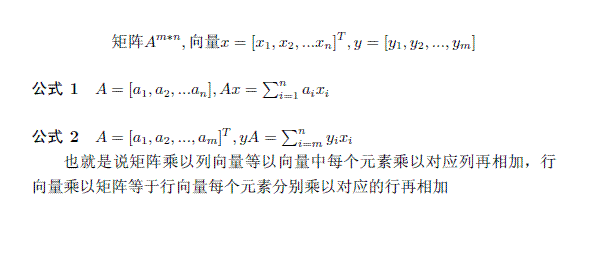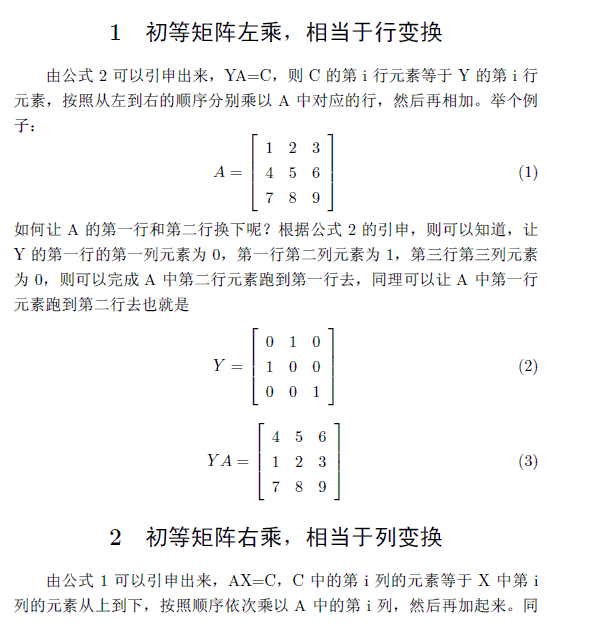针对于上图简单的理解为，初等行变换后所得到C，向量中的每个元素分别从左向右乘以矩阵中各行的元素，然后再相加，这个描述稍有一点点误解，可以直接以例子来说明，比如说y是3行2列，A是3行2列，那么初等行变换后C中第二行的结果，应该是个人浅薄理解，有错误请指正，谢谢


展开全文线性代数
• 初等矩阵和矩阵可逆性求解矩阵的逆矩阵的逆运算实现矩阵的逆 之前的笔记中曾提及过矩阵的逆的概念，但是并没有具体说明矩阵的逆的求法。 求解矩阵的逆 矩阵的逆运算 矩阵中AB=BA=IAB=BA=IAB=BA=I，则称B是A的逆矩阵...
初等矩阵和矩阵可逆性求解矩阵的逆矩阵的逆运算实现矩阵的逆初等矩阵矩阵的初等变换及初等矩阵初等矩阵和可逆性的关系矩阵可逆的重要性加速线性系统计算矩阵的逆相关重要命题
之前的笔记中曾提及过矩阵的逆的概念，但是并没有具体说明矩阵的逆的求法。
求解矩阵的逆
矩阵的逆运算
矩阵中$AB=BA=I$，则称B是A的逆矩阵，记为$B=A^{-1}$。只有方阵才有逆矩阵。
求解逆矩阵以下面的例子作为引入，因为Gauss-Jordan消元法是针对系数矩阵进行操作，而结果矩阵是随着系数矩阵的变化而变化的，所以可以将增广矩阵进一步变化，
$\begin{pmatrix}1&2&|&1&0\\3&4&|&0&1\end{pmatrix}\Longrightarrow\begin{pmatrix}1&0&|&x_{11}&x_{12}\\0&1&|&x_{21}&x_{22}\end{pmatrix}$
即左侧是系数矩阵，右侧是与之同形的单位矩阵，经过Gauss-Jordan消元法将左侧的系数矩阵化为行最简形式，如果化为行最简形式后左侧是一个单位矩阵，此时对应着唯一解的情况。上面的过程可以使用符号语言表示为，
$\begin{pmatrix}&A&|&I&\end{pmatrix}\Longrightarrow\begin{pmatrix}&I&|&A^{-1}&\end{pmatrix}$
矩阵的逆不可能存在无穷解，即一个方阵不可能存在无数个逆矩阵，只有存在一个逆矩阵或不存在逆矩阵这两种情况。

原因说明：
1.因为线性系统存在无穷解的情况可以看作是增广矩阵的最后一行为全0时对应的情况，但是求解逆矩阵时，增广矩阵右方是单位矩阵，不可能出现最下方的行为全0的情况。
2.当系数矩阵化为行最简形式后存在0行时，此时不存在逆矩阵。

上面的求解矩阵的逆的方式仅仅求取的是矩阵的右逆，与最开始矩阵的逆的定义相比，这看似是不严谨的。但是，另一个性质说明这种求取方式得到的结果是正确的，“如果一个方阵A有右逆B，则B也是A的左逆，即B是A的逆”。(以后给出证明)
实现矩阵的逆
求解矩阵的逆的过程本质上也是求解一个线性系统，只不过在一般的线性系统中，增广矩阵的右侧是一个列向量，而求逆时，增广矩阵的右侧是一个矩阵。所以依旧是在之前笔记的 LinearSystem类的基础上进行改造，
# -*- coding: utf-8 -*-
from .matrix import Matrix
from .vector import Vector
from ._global import EPSILON

class LinearSystem:
def __init__(self, A, b):
self._m = A.row_num()
self._n = A.col_num()
# 对构造方法进行如下修改
if isinstance(b, Vector):
self.Ab = [Vector(A.row_vector(i).underlying_list() + [b[i]]) for i in range(self._m)]
if isinstance(b, Matrix):
self.Ab = [Vector(A.row_vector(i).underlying_list() + b.row_vector(i).underlying_list() for i in range(self._m)]

self.pivots = []

def _forward(self):
......
def _backward(self):
......

# 对Gauss-Jordan消元法的返回值进行修改
def gauss_jordan_elimination(self):
self._forward()
self._backward()

# 检查系数矩阵为0时，结果矩阵不为0。此时是无解的情况
for i in range(len(self.pivots, self._m):
if not abs(self.Ab[i][-1]) < EPSILON:
return False
return True

def inv(A):
"""求解矩阵的逆"""
if A.row_num() != A.col_num():
return None
n = A.row_num()
ls = LinearSystem(A, Matrix.identity(n))
if not ls.gauss_jordan_elimination():
return None

invA = [[row[i] for i in range(n, 2*n)] for row in ls.Ab]

return Matrix(invA)


初等矩阵
矩阵的初等变换及初等矩阵
以该矩阵乘法进行说明，
$\begin{pmatrix}1&0&0\\0&1&0\\0&0&1\end{pmatrix}\cdot \begin{pmatrix}a&b&c\\d&e&f\\g&h&i\end{pmatrix}= \begin{pmatrix}a&b&c\\d&e&f\\g&h&i\end{pmatrix}\\$

矩阵的某一行乘以一个常数
$\begin{pmatrix}k&0&0\\0&1&0\\0&0&1\end{pmatrix}\cdot \begin{pmatrix}a&b&c\\d&e&f\\g&h&i\end{pmatrix}= \begin{pmatrix}k\cdot a&k\cdot b&k\cdot c\\d&e&f\\g&h&i\end{pmatrix}$
可总结为，
$\begin{pmatrix}k&0&0\\0&1&0\\0&0&1\end{pmatrix}$单位矩阵的某一行乘以一个常数。
矩阵的一行加(减)另一行
$\begin{pmatrix}1&0&0\\0&1&0\\1&0&1\end{pmatrix}\cdot \begin{pmatrix}a&b&c\\d&e&f\\g&h&i\end{pmatrix}= \begin{pmatrix}a&b&c\\d&e&f\\a+g&b+h&c+i\end{pmatrix}\\$
可总结为，
$\begin{pmatrix}1&0&0\\0&1&-p\\0&0&1\end{pmatrix}$单位矩阵的一行加(减)另一行的若干倍。
交换矩阵的两行
$\begin{pmatrix}1&0&0\\0&0&1\\0&1&0\end{pmatrix}\cdot \begin{pmatrix}a&b&c\\d&e&f\\g&h&i\end{pmatrix}= \begin{pmatrix}a&b&c\\g&h&i\\d&e&f\end{pmatrix}\\$
可总结为，
$\begin{pmatrix}1&0&0\\0&0&1\\0&1&0\end{pmatrix}$交换单位矩阵的两行。

如上这3种操作统称为“矩阵的初等变换”，任何形式的初等变换都可以用相应形式的矩阵进行表示，而这些用于表示初等变换的矩阵称为“初等矩阵”，通常记为$Ε$。
回顾之前的Gauss-Jordan消元法，把原矩阵化为行最简形式的过程实际上可以看作是寻找到一系列初等矩阵$Ε$，使得
$E_p\cdot ...\cdot E_3\cdot E_2\cdot E_1\cdot A=rref(A)$
初等矩阵和可逆性的关系
初等矩阵一定是可逆的。
因为初等变换是可逆的，所以初等矩阵也是可逆的。对于前一小节的初等矩阵，其对应的逆矩阵形式如下，
$\begin{pmatrix}k&0&0\\0&1&0\\0&0&1\end{pmatrix}\underrightarrow{\text{逆矩阵}}\begin{pmatrix}\frac{1}{k}&0&0\\0&1&0\\0&0&1\end{pmatrix}$
$\begin{pmatrix}1&0&0\\0&1&-p\\0&0&1\end{pmatrix}\underrightarrow{\text{逆矩阵}}\begin{pmatrix}1&0&0\\0&1&p\\0&0&1\end{pmatrix}$
$\begin{pmatrix}1&0&0\\0&0&1\\0&1&0\end{pmatrix}\underrightarrow{\text{逆矩阵}}\begin{pmatrix}1&0&0\\0&0&1\\0&1&0\end{pmatrix}$
回顾Gauss-Jordan消元过程求取矩阵的逆，
$E_p\cdot ...\cdot E_3\cdot E_2\cdot E_1\cdot A=rref(A)=I$
如果矩阵$A$可逆，则存在一系列初等矩阵$E$，使得
$E_p\cdot ...\cdot E_3\cdot E_2\cdot E_1\cdot A\cdot A^{-1}=I\cdot A^{-1}$
$E_p\cdot ...\cdot E_3\cdot E_2\cdot E_1\cdot I=A^{-1}$
即，
$\begin{pmatrix}&A&|&I&\end{pmatrix}\Longrightarrow\begin{pmatrix}&I&|&A^{-1}&\end{pmatrix}$
矩阵可逆的重要性
加速线性系统计算
对于线性系统$Ax=b$，有如下推导，
$A^{-1}\cdot A\cdot x=A^{-1}\cdot b\\x=A^{-1}\cdot b$
当线性系统的系数矩阵A不变，b会变化的情况下，使用矩阵的逆进行计算会大大加快计算速度。
矩阵的逆相关重要命题
矩阵的逆与很多重要的命题相关，这一系列的命题都是等价的。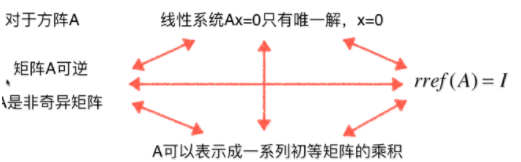对于上面诸多问题的证明，逐一证明时间太长，最好的方式是将四个命题串成串来证明，如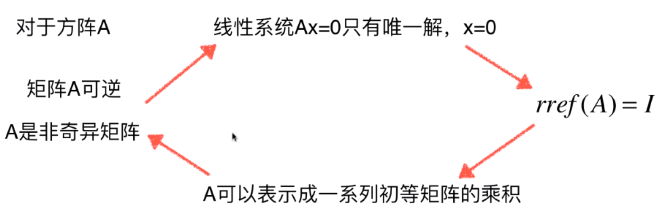顺时针方向证明各个命题，

证明：对于方阵$A$，若$A$可逆（是非奇异矩阵），则当线性系统 $Ax=0$时，仅有唯一解 $x=0$
$A\cdot x=0$
两端同乘$A^{-1}$，得到
$A^{-1}\cdot A\cdot x=A^{-1}\cdot0\\I\cdot x=0\\x=0$
证明：若线性系统 $Ax=0$只有唯一的0解，则$A$的行最简形式 $rref(A)=I$
因为$A$是n*n的矩阵，且$Ax=0$有唯一解，则有$n$个未知数，且$reff(A)$存在$n$个非0行
根据行最简形式的定义：$rref(A)=I$
证明：若矩阵$A$的行最简形式$rref(A)=I$，则$A$可以表示成一系列初等矩阵的乘积
依据上面的表述可写出，
$E_p\cdot ...\cdot E_3\cdot E_2\cdot E_1\cdot A=rref(A)=I$
等式两端同乘一连串逆矩阵，
$E_1^{-1}\cdot E_2^{-1}\cdot E_3^{-1}\cdot ...\cdot E_p^{-1}\cdot E_p\cdot ...\cdot E_3\cdot E_2\cdot E_1\cdot A=E_1^{-1}\cdot E_2^{-1}\cdot E_3^{-1}\cdot ...\cdot E_p^{-1}\cdot I$
可得，
$A=E_1^{-1}\cdot E_2^{-1}\cdot E_3^{-1}\cdot ...\cdot E_p^{-1}$
证明：若矩阵$A$可以表示成一系列初等矩阵的乘积，则该矩阵可逆（是非奇异矩阵）
$A=E_1\cdot E_2\cdot E_3\cdot ...\cdot E_p$
假设存在一个矩阵$B$，满足
$B=E_p^{-1}\cdot ...\cdot E_3^{-1}\cdot E_2^{-1}\cdot E_1^{-1}$
有矩阵的逆的运算性质，$B$可以表示为，
$B=(E_1\cdot E_2\cdot E_3\cdot ...\cdot E_p)^{-1}$
计算可得，
$A\cdot B=I\\B\cdot A=I$
故，$A$是可逆矩阵，其逆矩阵为$B$。

由此整个环证明完成，随便抽取出两个命题，都可以间接地通过环得出这两个命题是等价的。同时这四个命题的否命题也是等价的。

所谓两个事物“等价”可以很简单的理解为，说其中一件就等同于说另一件。在上面的四个命题中，提及矩阵可逆，则等同于又说了其他三个命题。


展开全文• 这篇文章和大家聊聊矩阵初等变换和矩阵的秩。矩阵初等变换这个概念可能在很多人听来有些陌生，但其实我们早在初中的解多元方程组的时候就用过它。只不过在课本当中，这种方法叫做消元法。我们先来看一个课本里的...
这篇文章和大家聊聊矩阵的初等变换和矩阵的秩。矩阵的初等变换这个概念可能在很多人听来有些陌生，但其实我们早在初中的解多元方程组的时候就用过它。只不过在课本当中，这种方法叫做消元法。我们先来看一个课本里的例子：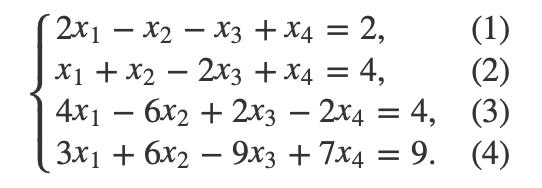假设我们要解这个方程，怎么做呢？首先，我们把(1)式加到(2)式，把(4)式加到(3)式，把(1)式乘6加到(4)式可以得到：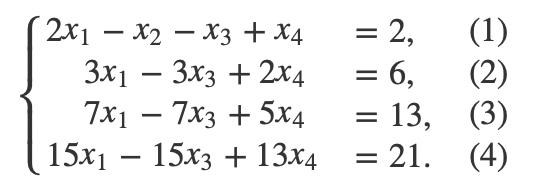我们再把(4)式减去(2)式乘5，可以解出x4=−3：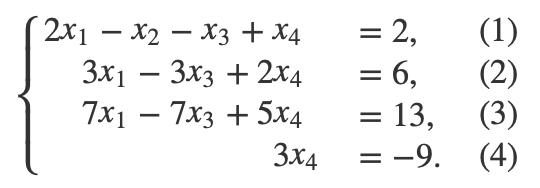我们把x4=−3带入，可以解出x1, x2, x3。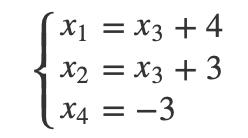因为消元之后，方程组的数量少于变量的数量，我们无法解出所有的变量。其中的x3可以取任何值。上面这个计算的方法我们都非常熟悉，如果我们用一个矩阵来表示所有的次数，那么这个矩阵D可以写成：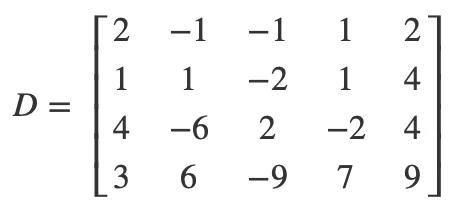那么，我们刚才消元的过程，其实就是对这个矩阵做初等变换。我们把这个过程总结一下，矩阵的初等变换操作包含以下三种：对调两行以数k,k≠0乘上某行的所有元素以数k,k≠0乘上某行所有元素并加到另一行以上的三种都是针对行为单位的，因此上面的三种变换也称为“行变换”。同样我们也可以对列做如上的三种操作，称为“列变换”。行变换和列变换结合就是矩阵的初等变换。同样，我们可以对D这个矩阵使用刚才我们上述的初等变换操作，将它变成如下这个结果：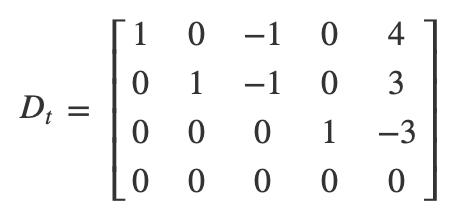它就对应方程组：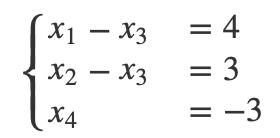Dt矩阵是经过初等行变换的结果，我们还可以再对它进行列变换，将它变得更简单，我们只要交换第三和第三列，之后就可以通过初等列变换把第五列消除，之后它就变成了下面这个样子：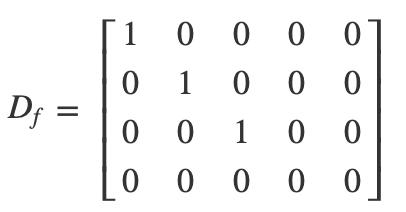我们用数据归纳法可以很容易证明，所有的m*n的矩阵经过一系列初等变换，都可以变成如下的形式：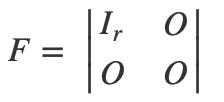r就是最简矩阵当中非零行的行数，它也被称为矩阵的秩。我们把A矩阵的秩记作: R(A)之前我们在介绍行列式的时候说过，行列式还存在多种性质。其中之一就是一个矩阵经过初等变换，它的行列式保持不变。我们又知道，如果行列式当中存在某一行或者某一列全部为0，那么它的行列式为0。所以，我们可以得到，对于n阶矩阵A而言，如果它的秩R(A)再根据我们前文当中有关可逆矩阵的定义，可以得到，可逆矩阵的秩就等于矩阵的阶数，不可逆矩阵的秩小于矩阵的阶数。所以，可逆矩阵又称为满秩矩阵，不可逆矩阵(奇异矩阵)又称为降秩矩阵。之前我们在复习行列式以及逆矩阵的时候，总觉得少了些什么，现在有了矩阵的秩的概念之后，这些知识就能串起来了。代码计算同样，numpy当中也继承了计算矩阵秩的工具。我们可以很轻松的用一行代码算出矩阵的秩，这样我们在判断矩阵是否可逆的时候，就不需要通过行列式来判断了。因为矩阵秩的计算要比行列式的计算快得多。import numpy as npnp.linalg.matrix_rank(a)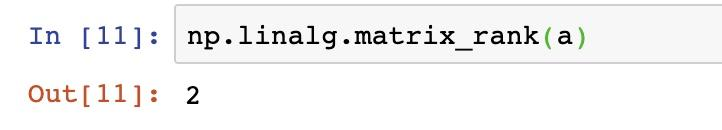有了矩阵秩的概念之后，我们后续的很多内容介绍起来都方便了许多，它也是矩阵领域当中非常重要的概念之一。线性方程组的解我们理解了矩阵的秩的概念之后，我们现学现用，看看它在线性方程组上的应用。我们之前在介绍行列式的时候，曾经介绍过n元n个等式的方程组的解，可以用行列式表示。但是现实当中我们遇见的方程组并不一定是n元n等式的，我们推广到一般的情况来看。假设当下有一个n元m个等式的方程组：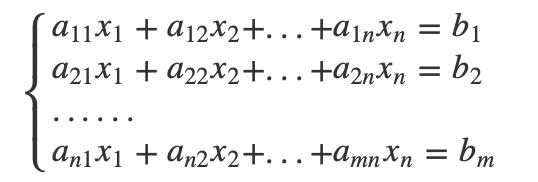我们可以将它写成矩阵相乘的形式：Ax = b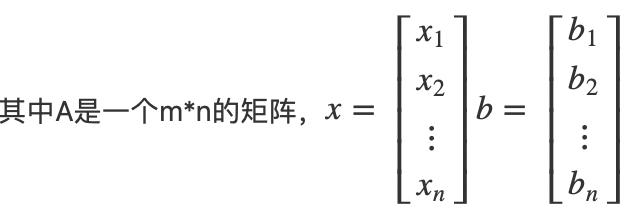我们利用系数矩阵A和增广矩阵B=(A,b)的秩，可以和方便地看出线性方程组是否有解。我们先来看结论：当R(A) < R(B)时无解当R(A) = R(B) = n时，有唯一解当R(A) = R(B) < n时，有无数解证明的过程也很简单，主要就是利用矩阵秩和最简矩阵的定义。我们假设R(A)=r，并将B矩阵化简成最简形式，假设得到的结果是：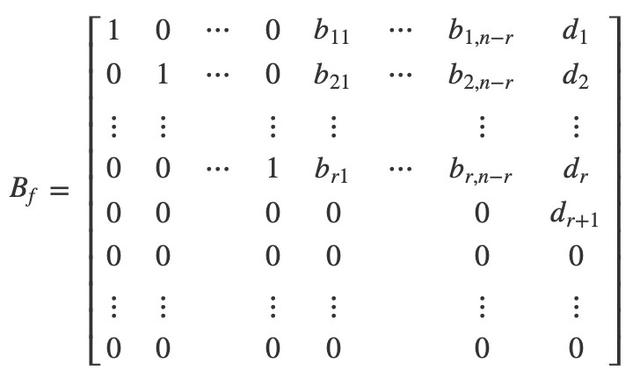(1) 显然，当R(A) < R(B)时，那么矩阵Bf中的dr+1 = 1，那么第r + 1行对应的方程0 = 1矛盾，所以方程无解。(2) 如果R(A) = R(B) = r = n，那么矩阵Bf中的dr+1 = 0，并且 bij都不出现，所以我们可以直接写出方程组的解：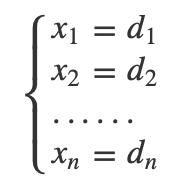此时，方程组有唯一解(3) 如果R(A) = R(B) = r < n，则B中的dr+1=0，我们写出对应的解：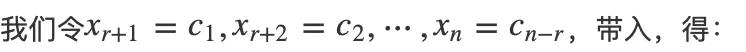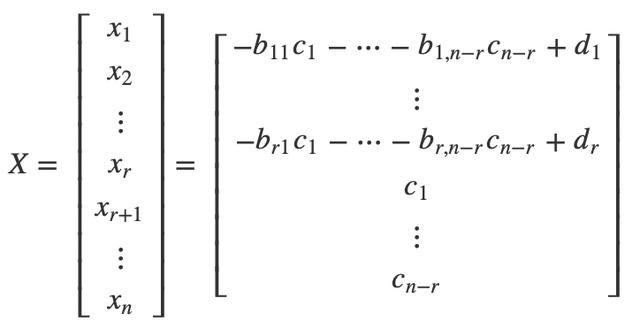由于参数c1, c2, ... cn-r可以取任意值，所以方程有无数解。上面写出的解的形式即是线性方程组的通解。齐次线性方程组如果我们将上面的线性方程组的常数项都置为0，就称为齐次线性方程组，如下：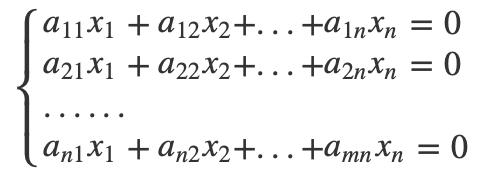齐次方程组最大的特点就是当x=0时一定有解，称为方程组的零解。我们还通过增广矩阵来判断，写出来其实还是刚才一样的形式：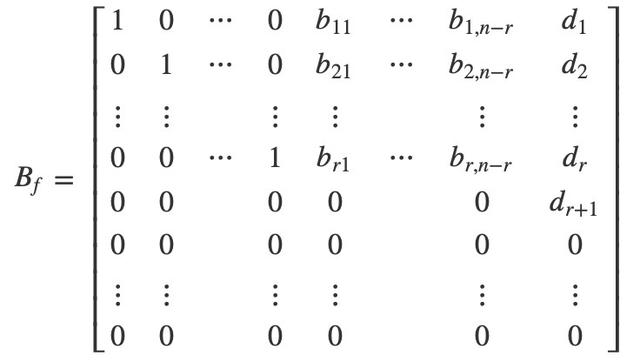和非齐次线性方程组不同的是，我们可以断定dr+1=0，如此一来就不存在无解的情况。这个时候我们要判断的就是方程组是否存在非零解，我们一样通过矩阵的秩来判断，判断的条件也很简单，如果R(A) = n，则不存在非零解，如果R(A) < n，则存在无数组非零解。我们先写出R(A) = n的情况，这时候的矩阵Bf为：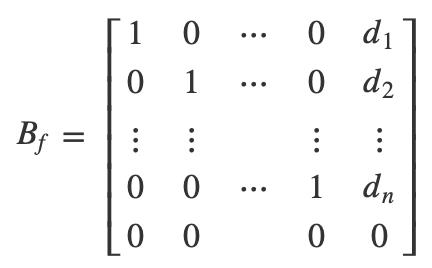也就是说：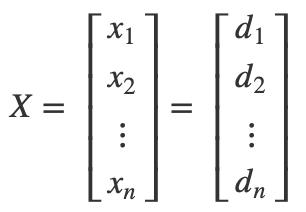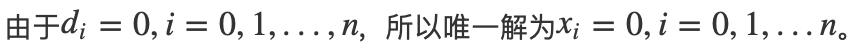当R(A) < n时方程组和非齐次方程组类似，唯一不同的是可以确定di=0 (i=1, 2, ...n)，我们直接带入之前的通项公式，可以得到：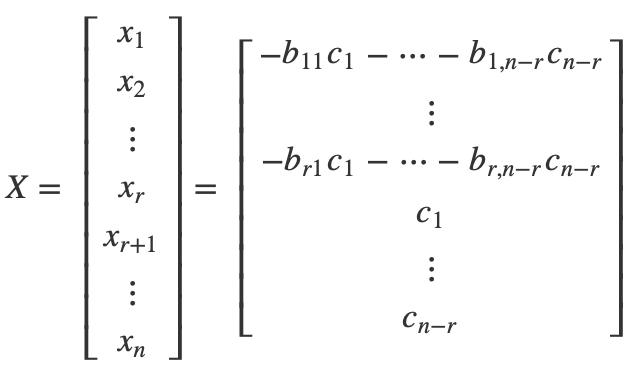线性方程组的解的公式和计算本身其实并不重要。因为在实际的算法领域，用到的也不多。但是理解线性方程组对于理解后面的向量以及线性空间非常有帮助，文中的公式看着恐怖，但冷静下来真的去试着理解一下，会发现也就那么回事。衷心希望大家学有收获，如果喜欢本文，请顺手给个关注吧~
展开全文• 矩阵初等变换这个概念可能在很多人听来有些陌生，但其实我们早在初中的解多元方程组的时候就用过它。只不过在课本当中，这种方法叫做消元法。我们先来看一个课本里的例子：假设我们要解这个方程，怎么做呢？首先，...矩阵的初等变换这个概念可能在很多人听来有些陌生，但其实我们早在初中的解多元方程组的时候就用过它。只不过在课本当中，这种方法叫做消元法。我们先来看一个课本里的例子：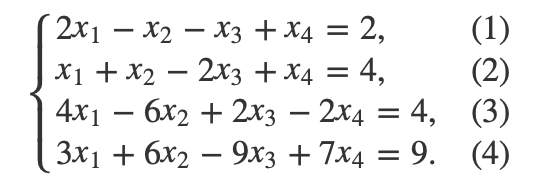假设我们要解这个方程，怎么做呢？首先，我们把(1)式加到(2)式，把(4)式加到(3)式，把(1)式乘6加到(4)式可以得到：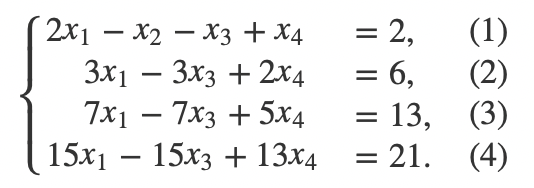我们再把(4)式减去(2)式乘5，可以解出x4=−3：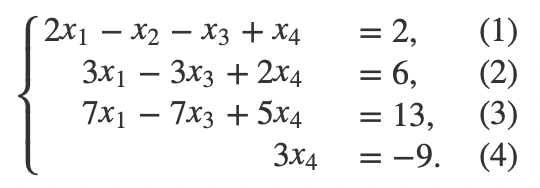我们把x4=−3带入，可以解出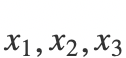。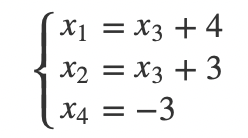因为消元之后，方程组的数量少于变量的数量，我们无法解出所有的变量。其中的可以取任何值。上面这个计算的方法我们都非常熟悉，如果我们用一个矩阵来表示所有的次数，那么这个矩阵D可以写成：那么，我们刚才消元的过程，其实就是对这个矩阵做初等变换。我们把这个过程总结一下，矩阵的初等变换操作包含以下三种：对调两行以数k,k≠0乘上某行的所有元素以数k,k≠0乘上某行所有元素并加到另一行以上的三种都是针对行为单位的，因此上面的三种变换也称为“行变换”。同样我们也可以对列做如上的三种操作，称为“列变换”。行变换和列变换结合就是矩阵的初等变换。同样，我们可以对D这个矩阵使用刚才我们上述的初等变换操作，将它变成如下这个结果：它就对应方程组：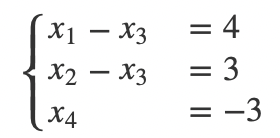Dt矩阵是经过初等行变换的结果，我们还可以再对它进行列变换，将它变得更简单，我们只要交换第三和第三列，之后就可以通过初等列变换把第五列消除，之后它就变成了下面这个样子：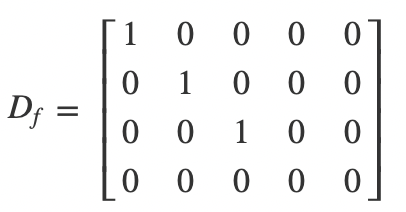我们用数据归纳法可以很容易证明，所有的m*n的矩阵经过一系列初等变换，都可以变成如下的形式：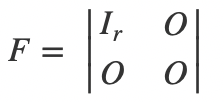r就是最简矩阵当中非零行的行数，它也被称为矩阵的秩。我们把A矩阵的秩记作: R(A)之前我们在介绍行列式的时候说过，行列式还存在多种性质。其中之一就是一个矩阵经过初等变换，它的行列式保持不变。我们又知道，如果行列式当中存在某一行或者某一列全部为0，那么它的行列式为0。所以，我们可以得到，对于n阶矩阵A而言，如果它的秩R(A)再根据我们前文当中有关可逆矩阵的定义，可以得到，可逆矩阵的秩就等于矩阵的阶数，不可逆矩阵的秩小于矩阵的阶数。所以，可逆矩阵又称为满秩矩阵，不可逆矩阵(奇异矩阵)又称为降秩矩阵。之前我们在复习行列式以及逆矩阵的时候，总觉得少了些什么，现在有了矩阵的秩的概念之后，这些知识就能串起来了。代码计算同样，numpy当中也继承了计算矩阵秩的工具。我们可以很轻松的用一行代码算出矩阵的秩，这样我们在判断矩阵是否可逆的时候，就不需要通过行列式来判断了。因为矩阵秩的计算要比行列式的计算快得多。import numpy as npnp.linalg.matrix_rank(a)有了矩阵秩的概念之后，我们后续的很多内容介绍起来都方便了许多，它也是矩阵领域当中非常重要的概念之一。线性方程组的解我们理解了矩阵的秩的概念之后，我们现学现用，看看它在线性方程组上的应用。我们之前在介绍行列式的时候，曾经介绍过n元n个等式的方程组的解，可以用行列式表示。但是现实当中我们遇见的方程组并不一定是n元n等式的，我们推广到一般的情况来看。假设当下有一个n元m个等式的方程组：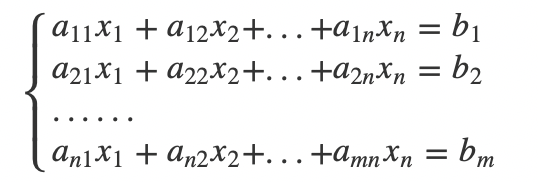我们可以将它写成矩阵相乘的形式：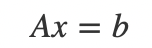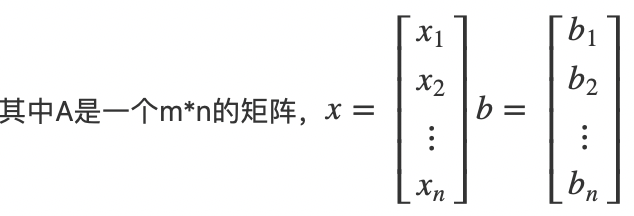我们利用系数矩阵A和增广矩阵B=(A,b)的秩，可以和方便地看出线性方程组是否有解。我们先来看结论：当R(A) < R(B)时无解当R(A) = R(B) = n时，有唯一解当R(A) = R(B) < n时，有无数解证明的过程也很简单，主要就是利用矩阵秩和最简矩阵的定义。我们假设R(A)=r，并将B矩阵化简成最简形式，假设得到的结果是：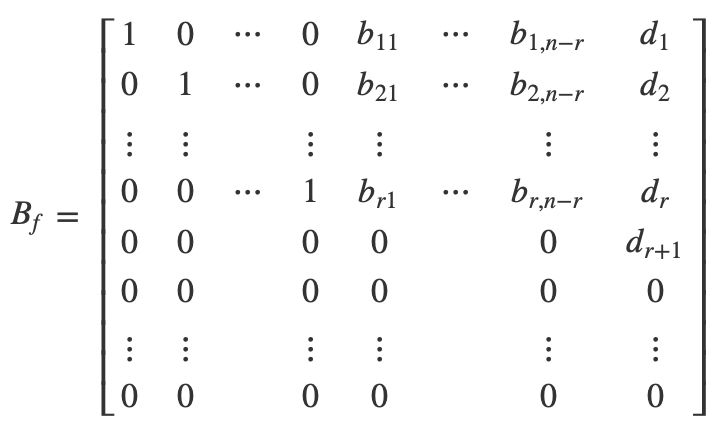(1)显然，当R(A) < R(B)时，那么矩阵中的，那么第r + 1行对应的方程0 = 1矛盾，所以方程无解。(2)如果R(A) = R(B) = r = n，那么矩阵中的，并且都不出现，所以我们可以直接写出方程组的解：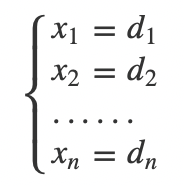此时，方程组有唯一解(3)如果R(A) = R(B) = r < n，则B中的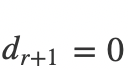，我们写出对应的解：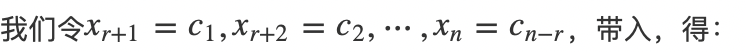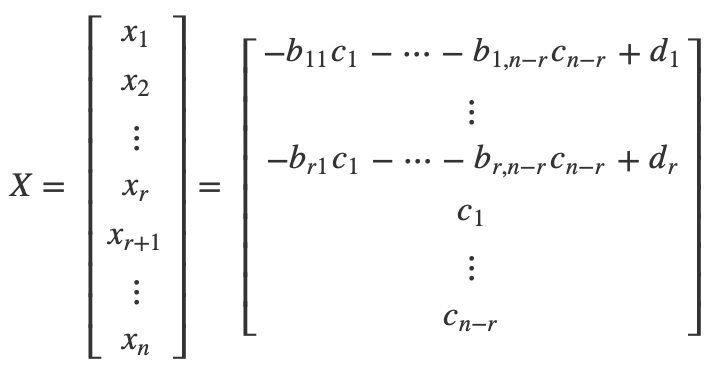由于参数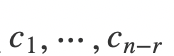可以取任意值，所以方程有无数解。上面写出的解的形式即是线性方程组的通解。齐次线性方程组如果我们将上面的线性方程组的常数项都置为0，就称为齐次线性方程组，如下：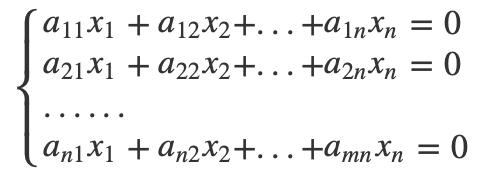齐次方程组最大的特点就是当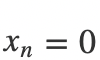时一定有解，称为方程组的零解。我们还通过增广矩阵来判断，写出来其实还是刚才一样的形式：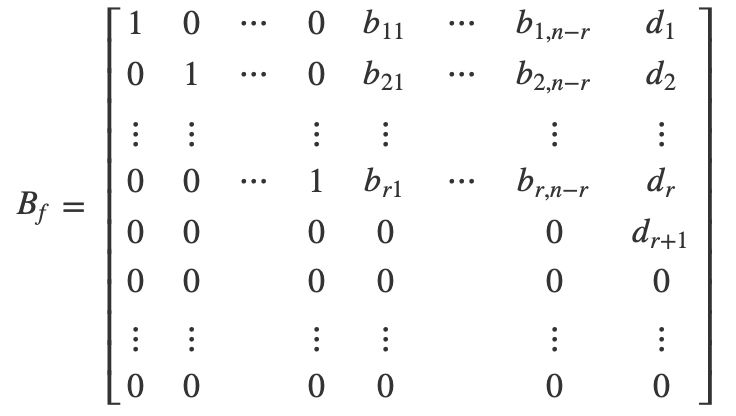和非齐次线性方程组不同的是，我们可以断定，如此一来就不存在无解的情况。这个时候我们要判断的就是方程组是否存在非零解，我们一样通过矩阵的秩来判断，判断的条件也很简单，如果R(A) = n，则不存在非零解，如果R(A) < n，则存在无数组非零解。我们先写出R(A) = n的情况，这时候的矩阵Bf为：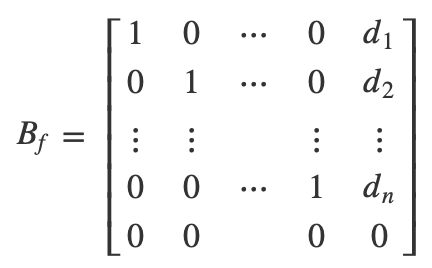也就是说：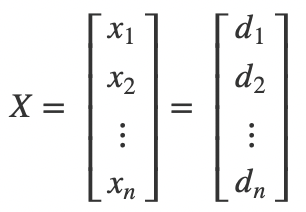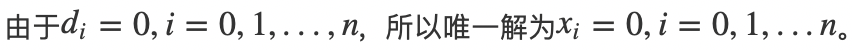当R(A) < n时方程组和非齐次方程组类似，唯一不同的是可以确定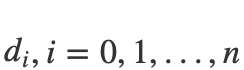，我们直接带入之前的通项公式，可以得到：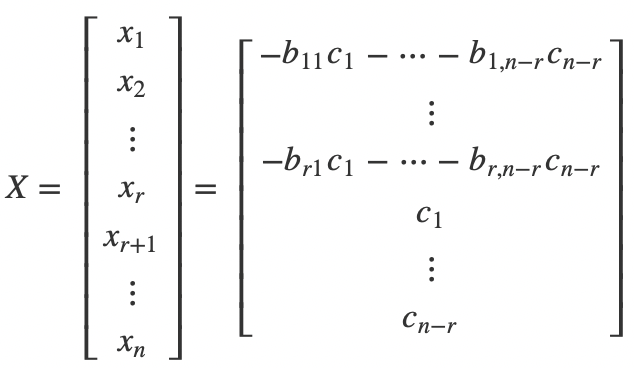线性方程组的解的公式和计算本身其实并不重要。因为在实际的算法领域，用到的也不多。但是理解线性方程组对于理解后面的向量以及线性空间非常有帮助，文中的公式看着恐怖，但冷静下来真的去试着理解一下，会发现也就那么回事。
展开全文• 这篇文章和大家聊聊矩阵初等变换和矩阵的秩。矩阵初等变换这个概念可能在很多人听来有些陌生，但其实我们早在初中的解多元方程组的时候就用过它。只不过在课本当中，这种方法叫做消元法。我们先来看一个课本里的...
• 这篇文章和大家聊聊矩阵初等变换和矩阵的秩。矩阵初等变换这个概念可能在很多人听来有些陌生，但其实我们早在初中的解多元方程组的时候就用过它。只不过在课本当中，这种方法叫做消元法。我们先来看一个课本里的...
• 这篇文章和大家聊聊矩阵初等变换和矩阵的秩。矩阵初等变换这个概念可能在很多人听来有些陌生，但其实我们早在初中的解多元方程组的时候就用过它。只不过在课本当中，这种方法叫做消元法。我们先来看一个课本里的...
• 矩阵初等变换这个概念可能在很多人听来有些陌生，但其实我们早在初中的解多元方程组的时候就用过它。只不过在课本当中，这种方法叫做消元法。我们先来看一个课本里的例子：假设我们要解这个方程，怎么做呢？首先，...
• 矩阵初等变换这个概念可能在很多人听来有些陌生，但其实我们早在初中的解多元方程组的时候就用过它。只不过在课本当中，这种方法叫做消元法。我们先来看一个课本里的例子： {2x1−x2−x3+x4=2,(1)x1+x2−2x3+x4=4,...线性代数 算法
• 1、矩阵初等变换 ...为了引进矩阵初等变换，先来分析用消元法解线性方程组的例子： 引例： {2x1−x2−x3+x4=2,x1+x2−2x3+x4=4,4x1−6x2+2x3−2x4=4.\begin{cases} 2x_1-x_2-x_3+x_4=2, \\ x_1+x_2-2x_3+x_...
• 应对n这个变量，可以使用矩阵初等变换来求解矩阵的可逆矩阵 矩阵初等变换 1） 消元法解线性方程组 先来看下这个例子，从x1∼x4x_1 \sim x_4x1​∼x4​ 和 4个方程，求解线性方程组 有这样一个方程组 {2x1−x2...AI 数学
• 展开全部给定三阶方阵A：A={{a,b,c},{d,e,f},{p,q,r}}，把第一e69da5e887aa62616964757a686964616f31333433633532行的第一个数字变成1，也就是用初等矩阵u来左乘A：u = {{1/a, 0, 0}, {0, 1, 0}, {0, 0, 1}}。...
• 第3章 矩阵初等变换与线性方程组 3.1矩阵初等变换 矩阵初等变换是一种...为引进矩阵初等变换，先来分析仪用消元法解线性方程组的例子初等变换定义 3.2 矩阵的秩 3.3 线性方程组的解 ...线性代数 线性方程组
• 求逆矩阵最有效的方法是初等变换法(虽然还有别的方法)。如果要求方阵 $$A$$ 的逆矩阵，标准的做法是：将矩阵 A 与单位矩阵 I 排成一个新的矩阵 (A I)；将此新矩阵 (A I) 做初等行变换，将它化成 (I B) 的形式若 A 是...
• 本篇笔记首先回顾了伴随...然后通过一个例子介绍了初等变换法求逆矩阵的过程，并对注意事项进行了总结；最后还讨论了通过初等变换判断矩阵可逆性、初等变换与行列式值的关系以及初等变换法求逆矩阵解题过程思路和总结。线性代数
• 1），其中 ： 求逆矩阵的方法有很多种，比如可以对 （ 为单位矩阵）进行初等行变换，得到 ，但鉴于对范德蒙德矩阵进行初等行变换是一件费力不讨好的事情，所以不建议采用。另一种方法是求线性方程组 的解，然后将 ...
• 举个例子，比如：A是一个3x4阶矩阵，假设它的最高阶子式为3阶 子式，那么A通过初等变化变成B之后，B的最高 阶子式同样为3阶子式，也就是初等变换不会改 变矩阵子式的阶数。3、矩阵秩的定义：...
• 一、用初等行变换化行最简形的技巧1. 一般是从左到右,一列一列处理2. 尽量避免分数的运算具体操作:1. 看本列中非零行的首非零元若有数a是其余数的公因子, 则用这个数把第本列其余的数消成零.2. 否则, 化出一个公因子...
• 在之前介绍的初等变换 只能 交换矩阵的两行。 例如 ，左乘矩阵 ==> 。 那如果想要交换矩阵的两列，需要右乘以置换矩阵。 ==> 综上，之前的例子中的问题就可以通过 再添加一个 置换矩阵 P’ 右乘 即可解决 ==&...线性代数
• 首先，对矩阵A通过初等行变换将其变为一个上三角矩阵。对于学习过线性代数的同学来说，这个过程应该很熟悉，线性代数考试中求行列式求逆一般都是通过这种方式来求解。然后，将原始矩阵A变为上三角矩阵的过程，对应的...
• 作者：苦逼的学生仔微信公众号ID：id1353695200矩阵的秩这知识的要求就是要理解：1、什么...举个例子，比如：A是一个3x4阶矩阵，假设它的最高阶子式为3阶子式，那么A通过初等变化变成B之后，B的最高阶子式同样为3阶...
• 1.3.1 矩阵的迹及其初等性质 1.3.2 矩阵的特征值及Gegorin圆盘定理 习 题 1.5第2章 线性代数基础 2.1 线性空间 2.1.1 线性空间的定义及例子 习 题 2.1 2.1.2 子空间的概念 习 题 2.2 2.1.3 基底和维数 习 题 2.3 ...
• 平面旋转群SO(2)是我们站在初等数学的角度就可以形成直观理解的，不平凡的例子。它易于理解、易于计算、理论完备。本文作为MP系列最初的讲座之一，力求让大家熟悉这个例子，并且于简单中挖掘其中丰富的代数结构，也...
• 如非奇异矩阵矩阵初等变换等。 高斯消元法 我们以一个例子来讲解高斯消元法 那么，我们可以将它抽象为一个增广矩阵， 该矩阵由系数矩阵和y矩阵组成。我们采用初等变换的方法将系数矩阵转换为上三角矩阵，该做法...
• 1、矩阵 A 可以分解为 L(上三角矩阵)、U(下三角矩阵，且对角线元素均为 1)注：上三角矩阵的对角线左下方的...例子 对于如下矩阵A，对A进行LU分解 首先将矩阵第一列对角线上元素A11下面的元素通过矩阵初等行变换变为学习笔记 机器学习
• 高斯消元可以通过初等行列变化把 增广矩阵 转换成 阶梯型矩阵，进而求解 n 个线性方程组的解，其时间复杂为 O(n^3^) 初等行列变换：(对一个方程组进行以下三个操作不会影响方程的解) 把某一行乘一个非0的数（方程...高斯消元 算法
• 第5章是模论，在其中用抽象代数的方法重新建立了矩阵的Jordan标准形理论。《抽象代数简明教程》编者长期从事代数学的教学工作，有丰富的教学经验。在处理数学问题时力求直接了当，努力做到叙述清楚，务求语言精确...
• 矩阵特征值与特征向量的计算 5.1 约化对称矩阵为对称三对角阵的豪斯荷尔德变换法 5.2 求对称三对角阵的全部特征值与特征向量 5.3 约化一般实矩阵为赫申伯格矩阵初等相似变换法 5.4 求赫身伯格矩阵全部...
• //进行初等行变换得到上三角矩阵 for (int i = j + 1; i ; i++) { s = gauss[i, j]; for (int m = j; m ; m++) { gauss[i, m] = Math.Round(gauss[i, m] - gauss[j, m] * s / gauss[j, j], 6); } } } //...c# 最小二乘法
• 虽然这些例子都是以C语言来编写的，但是算法并不局限于C语言。如果读者采用其他编程语言，例如C++、C#、VB、Java等，根据其语法格式进行适当的修改即可。 《C/C++常用算法手册》主要定位于有一定C／C++语言编程基础...C C++ 算法